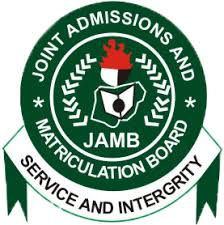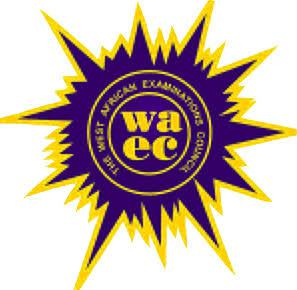vHomeJambWaecNecoNabtebNews
Welccome To Examloaded.Com>>>>  Nigeria's No 1 Exam Expo Website, With Over 7 Years of Experience Verified by Google #Na We Sure Pass!SUBSCRIBE HERE!!# 2019 JAMB Mathematics Syllabus (Maths) Questions & Answers

2019 Jamb Mathematics syllabus for Jamb Mathematics, The aim of the Unified Tertiary Matriculation Examination (UTME) syllabus in Mathematics is to prepare the candidates for the Board’s examination. It is designed to test the achievement of the course objectives, which are to:2019 Jamb Mathematics syllabus for Jamb Mathematics

(1) acquire computational and manipulative skills;
(2) develop precise, logical and formal reasoning skills;
(3) apply mathematical concepts to resolve issues in daily living; This syllabus is divided into five sections:
I. Number and Numeration.
II. Algebra
III. Geometry/Trigonometry.
IV. Calculus
V. Statistics

SECTION I: NUMBER AND
NUMERATION.
1. Number bases:
(a) operations in different number bases from 2 to 10;
(b) conversion from one base to another including fractional parts.
2. Fractions, Decimals, Approximations and Percentages:
(a) fractions and decimals
(b) significant figures
(c) decimal places
(d) percentage errors
(e) simple interest
(f) profit and loss per cent
(g) ratio, proportion and rate
3. Indices, Logarithms and Surds:
(a) laws of indices
(b) standard form
(c) laws of logarithm
(d) logarithm of any positive number to a given base.
(e) change of bases in logarithm and application.
Candidates should be able to:
i. perform four basic operations (x, ,-,÷);
ii. convert one base to another.
Candidates should be able to:
i. perform basic operations;
(x, ,-,÷) on fractions and decimals;
ii. express to specified number of significant figures and decimal places;
iii. calculate simple interest, profit and loss per cent, ratio proportion and rate.
Candidates should be able to:
i. apply the laws of indices in calculation;
ii. establish the relationship between indices and logarithms in solving problems;
iii. solve problems in different bases in logarithms.
iv. simplify and rationalize surds;
v. perform basic operations on surds
(f) relationship between indices and logarithm
(g) surds
4. Sets:
(a) types of sets
(b) algebra of sets
(c) venn diagrams and their applications.

2019 Jamb Mathematics syllabus for Jamb Mathematics

SECTIO N II: ALGEBRA
1. Poly nomials:
(a) change of subject of formula
(b) factor and remainder theorems
(c) factorization of polynomials of degree not exceeding 3.
(d) multiplication and division of polynomials
(e) roots of polynomials not exceeding degree 3
(f) simultaneous equations including one linear, one quadratic
(g) graphs of polynomials of degree not greater than 3
2. Varia tion:
(a) direct
(b) inverse
(c) joint
(d) partial
(e) percentage increase and decrease.
3. I nequalities:
(a) analytical and graphical solutions of linear inequalities.
(b) quadratic inequalities with integral roots only.
4. Progression:
(a) nth term of a progression
(b) sum of A. P. and G. P.
5. Binary Operations:
(a) properties of closure, commutativity, associativity and distributivity.
(b) identity and inverse elements.
Candidates should be able to:
i. identify types of sets, i.e empty, universal, compliments, subsets, finite, infinite and disjoint sets;
ii. solve set problems using symbol;
iii. use venn diagrams to solve problems involving not more than 3 sets.
Candidates should be able to:
i. find the subject of the formula of a given equation;
ii. apply factor and remainder theorem to factorize a given expression;
iii. multiply and divide polynomials of degree not more than 3;
iv. factorize by regrouping difference of two squares, perfect squares, etc.;
v. solve simultaneous equations – one linear, one quadratic;
vi. interpret graphs of polynomials including application to maximum and minimum values.
Candidates should be able to:
i. solve problems involving direct, inverse, joint and partial variations;
ii. solve problems on percentage increase and decrease in variation.
Candidates should be able to:
solve problems on linear and quadratic inequalities both analytically and graphically
Candidates should be able to:
i. determine the nth term of a progression;
ii. compute the sum of A. P. and G.P;
iii. sum to infinity a given G.P Candidates should be able to:
i. solve problems involving closure, commutativity, associativity and distributivity;
ii. solve problems involving identity and inverse elements.
6. Matrices and Determinants:
(a) algebra of matrices not exceeding 3 x 3.
(b) determinants of matrices not exceeding 3 x 3.
(c) inverses of 2 x 2 matrices
[excluding quadratic and higher degree equations].

2019 Jamb Mathematics syllabus for Jamb Mathematics

SECTION III: GEOMETRIC AND
TRIGONOMETRY
1. Euclidean Geometry:
(a) angles and lines
(b) polygon; triangles, quadrilaterals and general polygon.
(c) circles, angle properties, cyclic, quadrilaterals and intersecting chords.
(d) construction.
2. Mensuration:
(a) lengths and areas of plane geometrical figures.
(b) length s of arcs and chords of a circle.
(c) areas of sectors and segments of circles.
(d) surface areas and volumes of simple solids and composite figures.
(e) the earth as a sphere, longitudes and latitudes
3. Loci:
locus in 2 dimensions based on geometric principles relating to lines and curves.
4. Coordinate Geometry:
(a) midpoint and gradient of a line segment.
(b) distance between two points.
(c) parallel and perpendicular lines
(d) equations of straight lines.
Candidates should be able to:
i. perform basic operations (x, ,-,÷) on matrices;
ii. calculate determinants;
iii. compute inverses of 2 x 2 matrices
Candidates should be able to:
i. identify various types of lines and angles;
ii. solve problems involving polygons;
iii. calculate angles using circle theorems;
iv. identify construction procedures of special angles, e.g. 30°, 45°, 60°, 75°, 90°etc.
Candidates should be able to:
i. calculate the perimeters and areas of triangles, quadrilaterals, circles and composite figures;
ii. find the length of an arc, a chord and areas of sectors and segments of circles;
iii. calculate total surface areas and volumes of cuboids, cylinders. cones, pyramids, prisms, sphere and composite figures;
iv. determine the distance between two points on the earth’s surface.
Candidates should be able to:
identify and interpret loci relating to parallel lines, perpendicular bisectors, angle bisectors and circles.
Candidates should be able to:
i. determine the midpoint and gradient of a line segment;
ii. find distance between two points;
iii. identify conditions for parallelism and perpendicularity;
iv. find the equation of a line in the two-point form, point-slope form, slope intercept form and the general form.
5.Trigonometry:
(a) trigonometric ratios of angels.
(b) angles of elevation and depression and bearing.
(c) areas and solutions of triangle
(d) graphs of sine and cosine
(e) sine and cosine formulae.

2019 Jamb Mathematics syllabus for Jamb Mathematics

SECTI ON IV: CALCULUS
I. Differentiation:
(a) limit of a function;
(b) differentiation of explicit algebraic and simple trigonometric functions – sine, cosine and tangent.
2. Application of differentiation:
(a) rate of change
(b) maxima and minima
3. Integration:
(a) integration of explicit algebraic and simple trigonometric functions.
(a) area under the curve.
SECTION V: STATISTICS
1. Representation of data:
(a) frequency distribution
(b) histogram, bar chart and pie chart.
2. Measures of Location:
(a) mean, mode and median of ungrouped and grouped data – (simple cases only)
(b) cumulative frequency
Candidates should be able to:
i. calculate the sine, cosine and tarigent of angles between – 360°less than or equal to 0 (Zero) less than or equal to 360°;
ii. apply these special angles, e.g. 30°, 45°, 60°, 75°, 90°, 135°to solve simple problems in trigonometry;
iii. solve problems involving angles of elevation and depression and bearing;
iv. apply trigonometric formulae to find areas of triangles;
v. solve problems involving sine and cosine graphs.
Candidates should be able to:
i. find the limit of a function;
ii. differentiate explicit algebraic and simple trigonometric functions.
Candidates should be able to:
solve problems involving applications of rate of change, maxima and minima.
Candidates should be able to:
i. solve problems of integration involving algebraic and simple trigonometric functions;
ii. calculate area under the curve (simple cases only).
Candidates should be to:
i. identify and interpret frequency distribution tables;
ii. interpret information on histogram, bar chat and pie chart.
Candidates should be able to:
i. calculate the mean, mode and median of ungrouped and grouped data (simple cases only);
ii. use ogive to find the median quartiles and percentiles.
3. Measures of Dispersion:
range, mean deviation, variance and standard deviation.
4. Permutation and Combination
5. Probability

2019 Jamb Mathematics syllabus for Jamb Mathematics

Candidates should be able to:
calculate the range, mean deviation, variance and standard deviation of ungrouped and group data.
Candidates should be able to:
solve simple problems involving permutation and combination.
Candidates should be able to:
solve simple problems in probability (including addition and multiplication).
Adelodun A. A (2000). Distinction in Mathematics: Comprehensive Revision Text, (3rd Edition)
Anyebe, J. A. B (1998). Basic Mathematics for Senior Secondary Schools and Remedial Students in Higher/ institutions, Lagos: Kenny Moore.
Channon, J. B. Smith, A. M (2001). New General Mathematics for West Africa SSS 1 to 3, Lagos: Longman.
David -Osuagwu, M. name(s)? (2000). New School Mathematics for Senior Secondary Schools,
Onitsha: Africana – FIRST Publishers.
Egbe. E name(s)? (2000). Further Mathematics, Onitsha: Africana – FIRST Publishers
Ibude, S. O. name(s)? (2003). Agebra and Calculus for Schools and Colleges: LINCEL Publishers.
Tuttuh – Adegun M. R. name(s)? (1997). Further Mathematics Project Books 1 to 3, Ibadan: NPS Educational

That is all on 2019 Jamb Mathematics syllabus for Jamb Mathematics, feel free to ask Questions concerning the 2019 Jamb Mathematics syllabus for Jamb Mathematics using the comments section below.